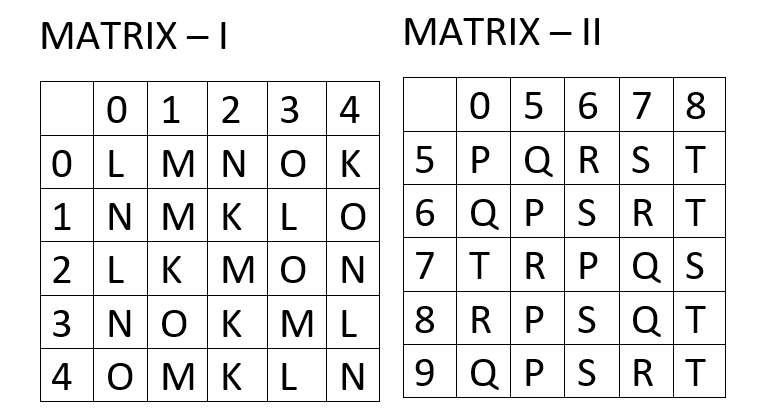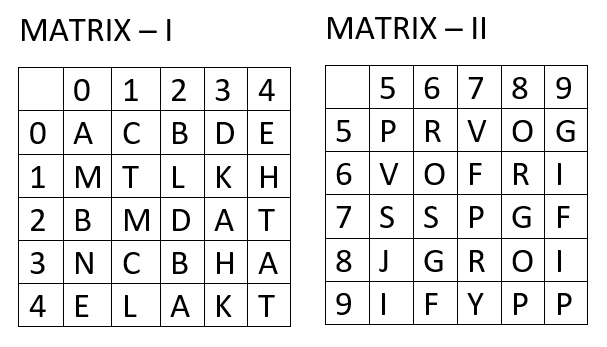## Q: A word is represented by only one set of numbers as given in any one of the Alternatives. The sets of numbers given in the alternatives are represented by two classes of alphabets as in two matrices given below. The columns and rows of Matrix are numbered from 0 to 4 and that of Matrix II are numbered from 5 to 9. A letter from these matrices can be represented first by its row and next by its column, e.g. 'N' can be represented by 02, 24 etc. and 'Q' can be represented by 56 , 78 etc. Similarly, you have to identify the set for the word 'SPORTS '.1 05df20424004abe5c78f9b0c0

• 1
67, 55, 31, 57, 69, 87
Correct
Wrong
• 2
58, 77, 20, 85, 79, 97
Correct
Wrong
• 3
24, 66, 40, 85, 89, 58
Correct
Wrong
• 4
87, 20, 23, 85, 75, 67
Correct
Wrong
• Workspace
• Discuss

• 1
7
Correct
Wrong
• 2
12
Correct
Wrong
• 3
4
Correct
Wrong
• 4
16
Correct
Wrong
• Workspace
• Discuss

## Q: In each of the following questions, which one of the four interchanges in signs and numbers would make the given equation correct? ( 3÷4 ) + 2=2  1 05df201a3a21bc06943856e29

• 1
+ and÷, 2 and 3
Correct
Wrong
• 2
+ and ÷, 2 and 4
Correct
Wrong
• 3
+ and ÷, 3 and 4
Correct
Wrong
• 4
No interchange , 3 and 4
Correct
Wrong
• Workspace
• Discuss

• 1
Evening
Correct
Wrong
• 2
Dark
Correct
Wrong
• 3
Day
Correct
Wrong
• 4
Dusk
Correct
Wrong
• Workspace
• Discuss

## Q: A word is represented by only one set of numbers as given in any one of the alternatives. The sets of numbers given in the alternatives are represented by two classes of alphabets as in two matrices given below. The columns and rows of Matrix I are numbered from 0 to 4 and that of Matrix II are numbered from 5 to 9. A letter from these matrices can be represented first by its row and next by its column, e. g. 'D' can be represented by 03, 22, etc. and 'R' can be represented by 56, 68, etc. Similarly, you have to identify the set for the word 'CAST’.8 05def5b336d799a05c00aa251

• 1
31, 42, 31, 20
Correct
Wrong
• 2
31, 00, 13, 20
Correct
Wrong
• 3
31, 12, 24, 00
Correct
Wrong
• 4
31, 00, 75, 44
Correct
Wrong
• Workspace
• Discuss

• 1
13th
Correct
Wrong
• 2
14th
Correct
Wrong
• 3
17th
Correct
Wrong
• 4
16th
Correct
Wrong
• 5
12th
Correct
Wrong
• Workspace
• Discuss

• 1
None
Correct
Wrong
• 2
One
Correct
Wrong
• 3
Three
Correct
Wrong
• 4
Two
Correct
Wrong
• 5
Five
Correct
Wrong
• Workspace
• Discuss

## Q: In a class, there are 80 students who study both Computer Science and Electronics. While 100 students study Computer Science, 120 Students study Electronics, How many of them study Computer Science only?  3 05def5bd52c071834c4fd2b47

• 1
100
Correct
Wrong
• 2
40
Correct
Wrong
• 3
120
Correct
Wrong
• 4
20
Correct
Wrong
• Workspace
• Discuss

Error Reported Successfully

Error Reported Successfully

Error Reported Successfully

Error Reported Successfully

Error Reported Successfully